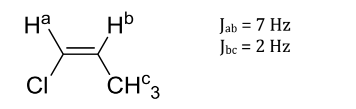# Problem: Use the following molecule and coupling constants for this problem. Draw the splitting tree that models the 1H NMR coupling, for Hb, based on the coupling constants (J) above. Label the coupling constants (in Hz) for each split of the peaks, just as they were labeled in your textbook.

###### Problem Details

Use the following molecule and coupling constants for this problem.

Draw the splitting tree that models the 1H NMR coupling, for Hb, based on the coupling constants (J) above. Label the coupling constants (in Hz) for each split of the peaks, just as they were labeled in your textbook.